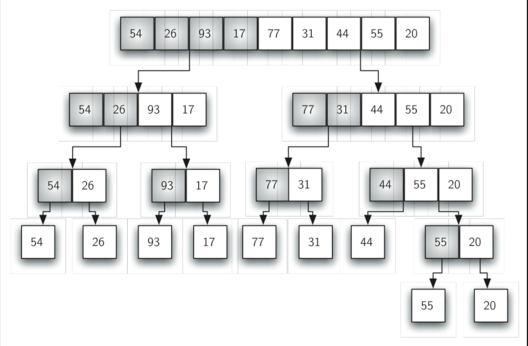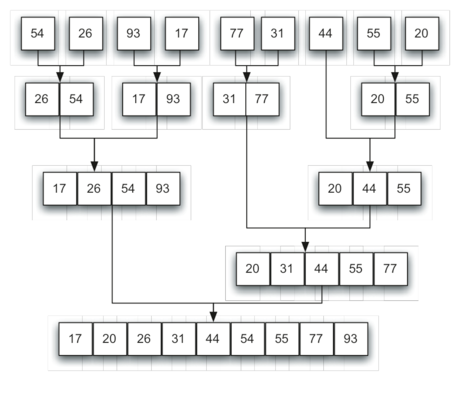# "considered the best sorting" algorithm

Classified in Computers

Written at onEnglish with a size of 223.75 KB.

 Tweet

Huffman coding is a lossless data compression algorithm. The idea is to assign variable-legth codes to input characters, lengths of the assigned codes are based on the frequencies of corresponding characters. The most frequent character gets the smallest code and the least frequent character gets the largest code

- In Huffman Algorithm, a set of nodes assigned with values is fed to the algorithm.
- Initially 2 nodes are considered and their sum forms their parent node.
- When a new element is considered, it can be added to the tree
- Its value and the previously calculated sum of the tree are used to form the new node which in turn becomes their parent.
- This algorithm is based on the frequency of occurrence of a data item.
- It uses a specific method for choosing the representation for each symbol.

Hash function

A hash function takes a group of characters (called a key) and maps it to a value of a certain length (called a hash value or hash). The hash value is representative of the original string of characters, but is normally smaller than the original.

Hashing is done for indexing and locating items in databases because it is easier to find the shorter hash value than the longer string. Hashing is also used in encryption.

This term is also known as a hashing algorithm or message digest function

One example of a hash function is called folding. This takes an original value, divides it into several parts, then adds the parts and uses the last four remaining digits as the hashed value or key.

Another example is called digit rearrangement. This takes the digits in certain positions of the original value, such as the third and sixth numbers, and reverses their order. It then uses the number left over as the hashed value

Merge Sort =Merge sort is a recursive algorithm follows the rule of�Divide and Conquer that continually splits a list in half. If the list is empty or has one item, it is sorted by definition (the base case). If the list has more than one item, we split the list and recursively invoke a merge sort on both halves. Once the two halves are sorted, the fundamental operation, called a�merge, is performed. Merging is the process of taking two smaller sorted lists and combining them together into a single, sorted new list.## Selection Sorting

Selection sorting is conceptually the most simplest sorting algorithm. This algorithm first finds the smallest element in the array and exchanges it with the element in the first position, then find the second smallest element and exchange it with the element in the second position, and continues in this way until the entire array is sorted.

#### How Selection Sorting Works

In the first pass, the smallest element found is 1, so it is placed at the first position, then leaving first element, smallest element is searched from the rest of the elements, 3 is the smallest, so it is then placed at the second position. Then we leave 1 and 3, from the rest of the elements, we search for the smallest and put it at third position and keep doing this, until array is sorted.

Radix Sort is an algorithm that sorts a list of numbers and comes under the category of distribution sort.
This sorting algorithm doesn't compare the numbers but distributes them, it works as follows:
1. Sorting takes place by distributing the list of number into a bucket by passing through the individual digits of a given number one-by-one beginning with the least significant part. Here, the number of buckets are a total of ten, which bare key values starting from 0 to 9.
2. After each pass, the numbers are collected from the buckets, keeping the numbers in order
3. Now, recursively redistribute the numbers as in the above step '1' but with a following reconsideration: take into account next most significant part of the number, which is then followed by above step '2'

heapsort is a comparison-based sorting algorithm. Heapsort can be thought of as an improved selection sort: like that algorithm, it divides its input into a sorted and an unsorted region, and it iteratively shrinks the unsorted region by extracting the largest element and moving that to the sorted region. The improvement consists of the use of a heap data structure rather than a linear-time search to find the maximum.

Although somewhat slower in practice on most machines than a well-implemented quicksort, it has the advantage of a more favorable worst-case O(n log n) runtime. Heapsort is an in-place algorithm, but it is not a stable sort.

## Algorithm

The heapsort algorithm involves preparing the list by first turning it into a max heap. The algorithm then repeatedly swaps the first value of the list with the last value, decreasing the range of values considered in the heap operation by one, and sifting the new first value into its position in the heap. This repeats until the range of considered values is one value in length.

The steps are:

1. Call the buildMaxHeap() function on the list. Also referred to as heapify(), this builds a heap from a list in O(n) operations.
2. Swap the first element of the list with the final element. Decrease the considered range of the list by one.
3. Call the siftDown() function on the list to sift the new first element to its appropriate index in the heap.
4. Go to step (2) unless the considered range of the list is one element.

The buildMaxHeap() operation is run once, and is O(n) in performance. The siftDown() function is O(log n), and is called n times. Therefore, the performance of this algorithm is O(n + n log n) = O(n log n).

The Address Calculation Sorting makes use of Hash Function Algorithm for sorting a set of elements. These types of functions are generally known as Order Preserving Hashing Function. This function is applied to all the elements to be sorted. According to the value of the hashing function, every element to be sorted is to be placed in a pre-defined set.

Every set is represented by a linked list. The starting address of each linked list can be maintained by an array of pointers. In every set, the elements have to be inserted in sorted order and, therefore, sorted linked lists needs to be taken.

General Search Tree

In computing,GiST( Generalized Search Tree), is a data structure and API that can be used to build a variety of disk-based search trees.

GiST is a generalization of the BST, providing a concurrent and recoverable height-balanced search tree infrastructure without making any assumptions about the type of data being stored, or the queries being serviced.

GiST is an example of software extensibility in the context of database systems: it allows the easy evolution of a database system to support new tree-based indexes.

General trees are those in which the number of sub trees for any node is not required to be 0, 1, or 2. The tree may be highly structured and therefore have 3 sub trees per node in which case it is called a ternary tree. However, it is often the case that the number of sub trees for any node may be variable. Some nodes may have 1 or no sub trees , others may have 3, some 4, or any other combination. The ternary tree is just a special case of a general tree (as is true of the binary tree).

Hashing is a technique that is used to uniquely identify a specific object from a group of similar objects. Some examples of how hashing is used in our lives include:

• In universities, each student is assigned a unique roll number that can be used to retrieve information about them.
• In libraries, each book is assigned a unique number that can be used to determine information about the book, such as its exact position in the library or the users it has been issued to etc.    In both these examples the students and books were hashed to a unique number.

In hashing, large keys are converted into small keys by using hash functions. The values are then stored in a data structure called hash table. The idea of hashing is to distribute entries (key/value pairs) uniformly across an array. Each element is assigned a key (converted key). By using that key you can access the element in O(1) time. Using the key, the algorithm (hash function) computes an index that suggests where an entry can be found or inserted.

Hashing is implemented in two steps:

1. An element is converted into an integer by using a hash function. This element can be used as an index to store the original element, which falls into the hash table.
2. The element is stored in the hash table where it can be quickly retrieved using hashed key.

hash = hashfunc(key)
index = hash % array_size

In this method, the hash is independent of the array size and it is then reduced to an index (a number between 0 and array_size − 1) by using the modulo operator (%).